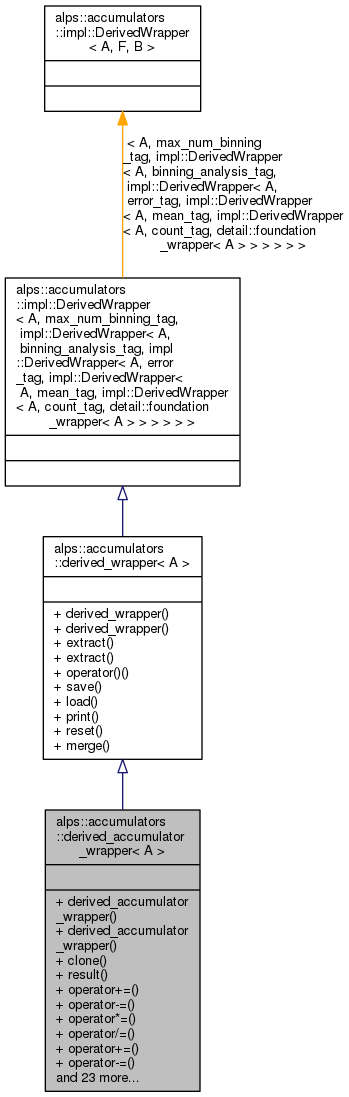ALPSCore reference
alps::accumulators::derived_accumulator_wrapper< A > Class Template Reference

#include <wrappers.hpp>

Inheritance diagram for alps::accumulators::derived_accumulator_wrapper< A >:Collaboration diagram for alps::accumulators::derived_accumulator_wrapper< A >:## Public Member Functions

derived_accumulator_wrapper ()

derived_accumulator_wrapper (A const &arg)

base_wrapper< typename value_type< A >::type > * clone () const

base_wrapper< typename value_type< A >::type > * result () const

void operator+= (base_wrapper< typename value_type< A >::type > const &)

void operator-= (base_wrapper< typename value_type< A >::type > const &)

void operator*= (base_wrapper< typename value_type< A >::type > const &)

void operator/= (base_wrapper< typename value_type< A >::type > const &)

void operator+= (long double)

void operator-= (long double)

void operator*= (long double)

void operator/= (long double)

void operator+= (wrapped_scalar_value_type)

void operator-= (wrapped_scalar_value_type)

void operator*= (wrapped_scalar_value_type)

void operator/= (wrapped_scalar_value_type)

void negate ()

void inverse ()

void sin ()

void cos ()

void tan ()

void sinh ()

void cosh ()

void tanh ()

void asin ()

void acos ()

void atan ()

void abs ()

void sqrt ()

void log ()

void sq ()

void cb ()

void cbrt ()Public Member Functions inherited from alps::accumulators::derived_wrapper< A >
derived_wrapper ()

derived_wrapper (A const &arg)

A & extract ()

A const & extract () const

void operator() (value_type const &value)

void save (hdf5::archive &ar) const

void load (hdf5::archive &ar)

void print (std::ostream &os, bool terse) const

void reset ()

void merge (const base_wrapper< value_type > &rhs)
Merge the given accumulator into this accumulator. More...

## Detailed Description

### template<typename A> class alps::accumulators::derived_accumulator_wrapper< A >

Definition at line 307 of file wrappers.hpp.

## Constructor & Destructor Documentation

template<typename A >
 alps::accumulators::derived_accumulator_wrapper< A >::derived_accumulator_wrapper ( )
inline

Definition at line 311 of file wrappers.hpp.

template<typename A >
 alps::accumulators::derived_accumulator_wrapper< A >::derived_accumulator_wrapper ( A const & arg )
inline

Definition at line 313 of file wrappers.hpp.

## Member Function Documentation

template<typename A >
 void alps::accumulators::derived_accumulator_wrapper< A >::abs ( )
inline

Definition at line 382 of file wrappers.hpp.

template<typename A >
 void alps::accumulators::derived_accumulator_wrapper< A >::acos ( )
inline

Definition at line 380 of file wrappers.hpp.

template<typename A >
 void alps::accumulators::derived_accumulator_wrapper< A >::asin ( )
inline

Definition at line 379 of file wrappers.hpp.

template<typename A >
 void alps::accumulators::derived_accumulator_wrapper< A >::atan ( )
inline

Definition at line 381 of file wrappers.hpp.

template<typename A >
 void alps::accumulators::derived_accumulator_wrapper< A >::cb ( )
inline

Definition at line 386 of file wrappers.hpp.

template<typename A >
 void alps::accumulators::derived_accumulator_wrapper< A >::cbrt ( )
inline

Definition at line 387 of file wrappers.hpp.

template<typename A >
 base_wrapper::type>* alps::accumulators::derived_accumulator_wrapper< A >::clone ( ) const
inline

Definition at line 315 of file wrappers.hpp.

template<typename A >
 void alps::accumulators::derived_accumulator_wrapper< A >::cos ( )
inline

Definition at line 374 of file wrappers.hpp.

template<typename A >
 void alps::accumulators::derived_accumulator_wrapper< A >::cosh ( )
inline

Definition at line 377 of file wrappers.hpp.

template<typename A >
 void alps::accumulators::derived_accumulator_wrapper< A >::inverse ( )
inline

Definition at line 364 of file wrappers.hpp.

template<typename A >
 void alps::accumulators::derived_accumulator_wrapper< A >::log ( )
inline

Definition at line 384 of file wrappers.hpp.

template<typename A >
 void alps::accumulators::derived_accumulator_wrapper< A >::negate ( )
inline

Definition at line 361 of file wrappers.hpp.

template<typename A >
 void alps::accumulators::derived_accumulator_wrapper< A >::operator*= ( base_wrapper< typename value_type< A >::type > const & )
inline

Definition at line 328 of file wrappers.hpp.

template<typename A >
 void alps::accumulators::derived_accumulator_wrapper< A >::operator*= ( long double )
inline

Definition at line 341 of file wrappers.hpp.

template<typename A >
 void alps::accumulators::derived_accumulator_wrapper< A >::operator*= ( wrapped_scalar_value_type )
inline

Definition at line 354 of file wrappers.hpp.

template<typename A >
 void alps::accumulators::derived_accumulator_wrapper< A >::operator+= ( base_wrapper< typename value_type< A >::type > const & )
inline

Definition at line 322 of file wrappers.hpp.

template<typename A >
 void alps::accumulators::derived_accumulator_wrapper< A >::operator+= ( long double )
inline

Definition at line 335 of file wrappers.hpp.

template<typename A >
 void alps::accumulators::derived_accumulator_wrapper< A >::operator+= ( wrapped_scalar_value_type )
inline

Definition at line 348 of file wrappers.hpp.

template<typename A >
 void alps::accumulators::derived_accumulator_wrapper< A >::operator-= ( base_wrapper< typename value_type< A >::type > const & )
inline

Definition at line 325 of file wrappers.hpp.

template<typename A >
 void alps::accumulators::derived_accumulator_wrapper< A >::operator-= ( long double )
inline

Definition at line 338 of file wrappers.hpp.

template<typename A >
 void alps::accumulators::derived_accumulator_wrapper< A >::operator-= ( wrapped_scalar_value_type )
inline

Definition at line 351 of file wrappers.hpp.

template<typename A >
 void alps::accumulators::derived_accumulator_wrapper< A >::operator/= ( base_wrapper< typename value_type< A >::type > const & )
inline

Definition at line 331 of file wrappers.hpp.

template<typename A >
 void alps::accumulators::derived_accumulator_wrapper< A >::operator/= ( long double )
inline

Definition at line 344 of file wrappers.hpp.

template<typename A >
 void alps::accumulators::derived_accumulator_wrapper< A >::operator/= ( wrapped_scalar_value_type )
inline

Definition at line 357 of file wrappers.hpp.

template<typename A >
 base_wrapper::type>* alps::accumulators::derived_accumulator_wrapper< A >::result ( ) const
inline

Definition at line 318 of file wrappers.hpp.

template<typename A >
 void alps::accumulators::derived_accumulator_wrapper< A >::sin ( )
inline

Definition at line 373 of file wrappers.hpp.

template<typename A >
 void alps::accumulators::derived_accumulator_wrapper< A >::sinh ( )
inline

Definition at line 376 of file wrappers.hpp.

template<typename A >
 void alps::accumulators::derived_accumulator_wrapper< A >::sq ( )
inline

Definition at line 385 of file wrappers.hpp.

template<typename A >
 void alps::accumulators::derived_accumulator_wrapper< A >::sqrt ( )
inline

Definition at line 383 of file wrappers.hpp.

template<typename A >
 void alps::accumulators::derived_accumulator_wrapper< A >::tan ( )
inline

Definition at line 375 of file wrappers.hpp.

template<typename A >
 void alps::accumulators::derived_accumulator_wrapper< A >::tanh ( )
inline

Definition at line 378 of file wrappers.hpp.

The documentation for this class was generated from the following file: